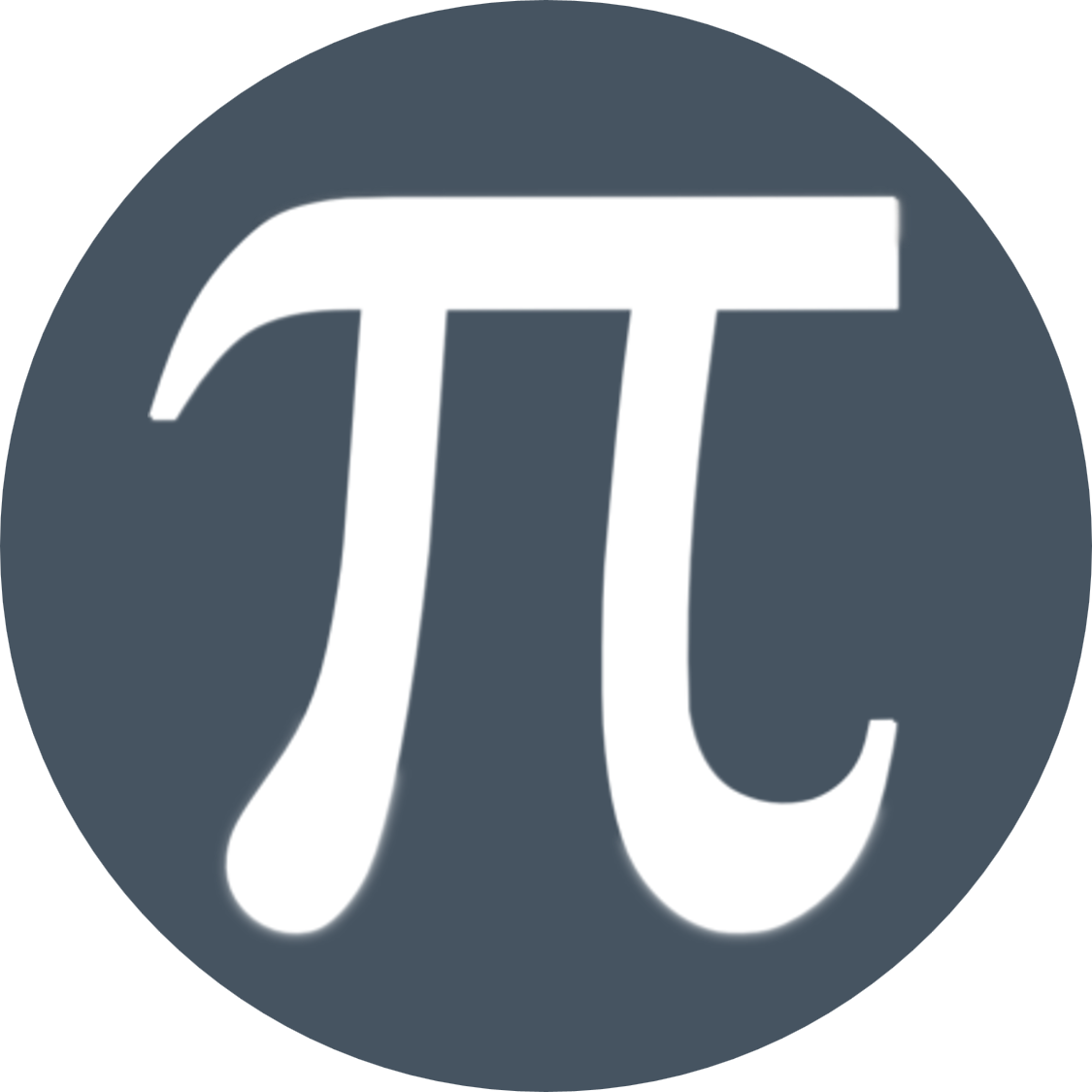# MathematicsMathematics is taught in 10 ability sets with students able to move between the sets depending on progress.

We aim to:

• provide learners with a rich experience of Mathematics
• provide learners with greater opportunities for using and interpreting Mathematics
• improve attitudes towards Mathematics
• inspire and prepare young people to continue Mathematics studies beyond GCSE

What resources are available?

A variety of materials are available in lessons with each student assigned a copy of the higher or foundation text book. Each student is also given access to the MyMaths web site which has a large database of lesson resources and worksheets.

## Foundation Curriculum

Year 10 Year 11
Aut.1 Angles, Scale diagrams, HCF/LCM, Algebra Probability, Shapes in 2D & 3D, Scatter graphs
Aut.2 Linear Graphs, Sequences, Circles Simultaneous equations, Graph sketching
Spr.1 Real life graphs, Equations, Indices & SIF Proportion, Pythagoras and Trigonometry
Sum.1 Percentages, Pythagoras, Loci Revision
Sum.2 2D representations, Statistical analysis

## Higher Curriculum

Year 10 Year 11
Aut.1 Angles, Algebra, Linear Graphs Algebra, Probability
Aut.2 Approximation, Sequences, Circles, Compound shapes Graph sketching, proportion, Inequalities
Spr.1 Real life graphs, Equations, Indices & SIF, Surds Vectors, Transformation of functions, Eqn of circle
Spr.2 Standard Index form, Measures, Transformations Circle theorems, Algebraic fractions, Non RAT trig
Sum.1 Percentages, Loci, Congruence Pre-Calculus and Revision
Sum.2 Statistical Analysis and Algebra

How is it assessed?

Students follow either the linear Higher or linear Foundation AQA syllabuses. Students sit three exam papers, one non-calculator and two calculator papers, all of which are taken at the end of Year 11. Each paper is marked out of 80 and is worth 33% of the exam.

There is no coursework in Mathematics.

## Career Pathways

Many students go on to study Mathematics at A Level and beyond at university. Mathematics is a highly respected qualification that demonstrates the ability to think logically and solve problems.

Sixth Forms, colleges and employers require either a grade 4 or 5 or above in Mathematics. We guide pupils towards the route that is most likely to deliver this outcome.

Career Options

You can watch a great series of videos of young people who are working in jobs that use maths on the BBC Bitesize website.